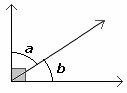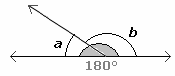The word "adjacent" means "next" or "neighboring"

Adjacent angles are angles just next to each other. Adjacent angles share a common vertex and a common side, but do not overlap.

If two adjacent angles form a right angle (90o), then they are complementaryIf two adjacent angles form a straight angle (180o), then they are supplementary.In the figure shown, a and b are adjacent angles. They have a common vertex O and a common side OA.Name all pairs of angles in the diagram that are adjacent.Solution:

Adjacent angles are angles just next to each other. Adjacent angles share a common vertex and a common side, but do not overlap.
∠a and ∠b,
∠b and ∠c
∠c and ∠d
∠d and ∠e
∠e and ∠a are the pairs of adjacent angels in the diagram shown above.